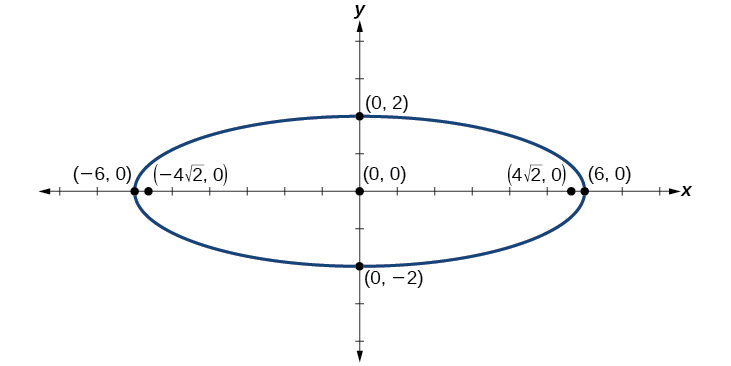# 12.1 The ellipse  (Page 5/16)

 Page 5 / 16

What is the standard form equation of the ellipse that has vertices $\text{\hspace{0.17em}}\left(-3,3\right)\text{\hspace{0.17em}}$ and $\text{\hspace{0.17em}}\left(5,3\right)\text{\hspace{0.17em}}$ and foci $\text{\hspace{0.17em}}\left(1-2\sqrt{3},3\right)\text{\hspace{0.17em}}$ and $\text{\hspace{0.17em}}\left(1+2\sqrt{3},3\right)?$

$\frac{{\left(x-1\right)}^{2}}{16}+\frac{{\left(y-3\right)}^{2}}{4}=1$

## Graphing ellipses centered at the origin

Just as we can write the equation for an ellipse given its graph, we can graph an ellipse given its equation. To graph ellipses centered at the origin, we use the standard form for horizontal ellipses and for vertical ellipses.

Given the standard form of an equation for an ellipse centered at $\text{\hspace{0.17em}}\left(0,0\right),$ sketch the graph.

1. Use the standard forms of the equations of an ellipse to determine the major axis, vertices, co-vertices, and foci.
1. If the equation is in the form $\text{\hspace{0.17em}}\frac{{x}^{2}}{{a}^{2}}+\frac{{y}^{2}}{{b}^{2}}=1,\text{\hspace{0.17em}}$ where $\text{\hspace{0.17em}}a>b,\text{\hspace{0.17em}}$ then
• the major axis is the x -axis
• the coordinates of the vertices are $\text{\hspace{0.17em}}\left(±a,0\right)$
• the coordinates of the co-vertices are $\text{\hspace{0.17em}}\left(0,±b\right)$
• the coordinates of the foci are $\text{\hspace{0.17em}}\left(±c,0\right)$
2. If the equation is in the form $\text{\hspace{0.17em}}\frac{{x}^{2}}{{b}^{2}}+\frac{{y}^{2}}{{a}^{2}}=1,$ where $\text{\hspace{0.17em}}a>b,\text{\hspace{0.17em}}$ then
• the major axis is the y -axis
• the coordinates of the vertices are $\text{\hspace{0.17em}}\left(0,±a\right)$
• the coordinates of the co-vertices are $\text{\hspace{0.17em}}\left(±b,0\right)$
• the coordinates of the foci are $\text{\hspace{0.17em}}\left(0,±c\right)$
2. Solve for $\text{\hspace{0.17em}}c\text{\hspace{0.17em}}$ using the equation $\text{\hspace{0.17em}}{c}^{2}={a}^{2}-{b}^{2}.$
3. Plot the center, vertices, co-vertices, and foci in the coordinate plane, and draw a smooth curve to form the ellipse.

## Graphing an ellipse centered at the origin

Graph the ellipse given by the equation, $\text{\hspace{0.17em}}\frac{{x}^{2}}{9}+\frac{{y}^{2}}{25}=1.\text{\hspace{0.17em}}$ Identify and label the center, vertices, co-vertices, and foci.

First, we determine the position of the major axis. Because $\text{\hspace{0.17em}}25>9,$ the major axis is on the y -axis. Therefore, the equation is in the form $\text{\hspace{0.17em}}\frac{{x}^{2}}{{b}^{2}}+\frac{{y}^{2}}{{a}^{2}}=1,$ where $\text{\hspace{0.17em}}{b}^{2}=9\text{\hspace{0.17em}}$ and $\text{\hspace{0.17em}}{a}^{2}=25.\text{\hspace{0.17em}}$ It follows that:

• the center of the ellipse is $\text{\hspace{0.17em}}\left(0,0\right)$
• the coordinates of the vertices are $\text{\hspace{0.17em}}\left(0,±a\right)=\left(0,±\sqrt{25}\right)=\left(0,±5\right)$
• the coordinates of the co-vertices are $\text{\hspace{0.17em}}\left(±b,0\right)=\left(±\sqrt{9},0\right)=\left(±3,0\right)$
• the coordinates of the foci are $\text{\hspace{0.17em}}\left(0,±c\right),\text{\hspace{0.17em}}$ where $\text{\hspace{0.17em}}{c}^{2}={a}^{2}-{b}^{2}\text{\hspace{0.17em}}$ Solving for $\text{\hspace{0.17em}}c,$ we have:

$\begin{array}{l}c=±\sqrt{{a}^{2}-{b}^{2}}\hfill \\ \text{\hspace{0.17em}}\text{\hspace{0.17em}}\text{\hspace{0.17em}}=±\sqrt{25-9}\hfill \\ \text{\hspace{0.17em}}\text{\hspace{0.17em}}\text{\hspace{0.17em}}=±\sqrt{16}\hfill \\ \text{\hspace{0.17em}}\text{\hspace{0.17em}}\text{\hspace{0.17em}}=±4\hfill \end{array}$

Therefore, the coordinates of the foci are $\text{\hspace{0.17em}}\left(0,±4\right).$

Next, we plot and label the center, vertices, co-vertices, and foci, and draw a smooth curve to form the ellipse. See [link] .

Graph the ellipse given by the equation $\text{\hspace{0.17em}}\frac{{x}^{2}}{36}+\frac{{y}^{2}}{4}=1.\text{\hspace{0.17em}}$ Identify and label the center, vertices, co-vertices, and foci.

center: $\text{\hspace{0.17em}}\left(0,0\right);\text{\hspace{0.17em}}$ vertices: $\text{\hspace{0.17em}}\left(±6,0\right);\text{\hspace{0.17em}}$ co-vertices: $\text{\hspace{0.17em}}\left(0,±2\right);\text{\hspace{0.17em}}$ foci: $\text{\hspace{0.17em}}\left(±4\sqrt{2},0\right)$## Graphing an ellipse centered at the origin from an equation not in standard form

Graph the ellipse given by the equation $\text{\hspace{0.17em}}4{x}^{2}+25{y}^{2}=100.\text{\hspace{0.17em}}$ Rewrite the equation in standard form. Then identify and label the center, vertices, co-vertices, and foci.

First, use algebra to rewrite the equation in standard form.

Next, we determine the position of the major axis. Because $\text{\hspace{0.17em}}25>4,\text{\hspace{0.17em}}$ the major axis is on the x -axis. Therefore, the equation is in the form $\text{\hspace{0.17em}}\frac{{x}^{2}}{{a}^{2}}+\frac{{y}^{2}}{{b}^{2}}=1,\text{\hspace{0.17em}}$ where $\text{\hspace{0.17em}}{a}^{2}=25\text{\hspace{0.17em}}$ and $\text{\hspace{0.17em}}{b}^{2}=4.\text{\hspace{0.17em}}$ It follows that:

• the center of the ellipse is $\text{\hspace{0.17em}}\left(0,0\right)$
• the coordinates of the vertices are $\text{\hspace{0.17em}}\left(±a,0\right)=\left(±\sqrt{25},0\right)=\left(±5,0\right)$
• the coordinates of the co-vertices are $\text{\hspace{0.17em}}\left(0,±b\right)=\left(0,±\sqrt{4}\right)=\left(0,±2\right)$
• the coordinates of the foci are $\text{\hspace{0.17em}}\left(±c,0\right),\text{\hspace{0.17em}}$ where $\text{\hspace{0.17em}}{c}^{2}={a}^{2}-{b}^{2}.\text{\hspace{0.17em}}$ Solving for $\text{\hspace{0.17em}}c,\text{\hspace{0.17em}}$ we have:

$\begin{array}{l}c=±\sqrt{{a}^{2}-{b}^{2}}\hfill \\ \text{\hspace{0.17em}}\text{\hspace{0.17em}}\text{\hspace{0.17em}}=±\sqrt{25-4}\hfill \\ \text{\hspace{0.17em}}\text{\hspace{0.17em}}\text{\hspace{0.17em}}=±\sqrt{21}\hfill \end{array}$

Therefore the coordinates of the foci are $\text{\hspace{0.17em}}\left(±\sqrt{21},0\right).$

Next, we plot and label the center, vertices, co-vertices, and foci, and draw a smooth curve to form the ellipse.

How look for the general solution of a trig function
stock therom F=(x2+y2) i-2xy J jaha x=a y=o y=b
root under 3-root under 2 by 5 y square
The sum of the first n terms of a certain series is 2^n-1, Show that , this series is Geometric and Find the formula of the n^th
cosA\1+sinA=secA-tanA
why two x + seven is equal to nineteen.
The numbers cannot be combined with the x
Othman
2x + 7 =19
humberto
2x +7=19. 2x=19 - 7 2x=12 x=6
Yvonne
because x is 6
SAIDI
what is the best practice that will address the issue on this topic? anyone who can help me. i'm working on my action research.
simplify each radical by removing as many factors as possible (a) √75
how is infinity bidder from undefined?
what is the value of x in 4x-2+3
give the complete question
Shanky
4x=3-2 4x=1 x=1+4 x=5 5x
Olaiya
hi can you give another equation I'd like to solve it
Daniel
what is the value of x in 4x-2+3
Olaiya
if 4x-2+3 = 0 then 4x = 2-3 4x = -1 x = -(1÷4) is the answer.
Jacob
4x-2+3 4x=-3+2 4×=-1 4×/4=-1/4
LUTHO
then x=-1/4
LUTHO
4x-2+3 4x=-3+2 4x=-1 4x÷4=-1÷4 x=-1÷4
LUTHO
A research student is working with a culture of bacteria that doubles in size every twenty minutes. The initial population count was  1350  bacteria. Rounding to five significant digits, write an exponential equation representing this situation. To the nearest whole number, what is the population size after  3  hours?
v=lbh calculate the volume if i.l=5cm, b=2cm ,h=3cm
Need help with math
Peya
can you help me on this topic of Geometry if l help you
litshani
( cosec Q _ cot Q ) whole spuare = 1_cosQ / 1+cosQ
A guy wire for a suspension bridge runs from the ground diagonally to the top of the closest pylon to make a triangle. We can use the Pythagorean Theorem to find the length of guy wire needed. The square of the distance between the wire on the ground and the pylon on the ground is 90,000 feet. The square of the height of the pylon is 160,000 feet. So, the length of the guy wire can be found by evaluating √(90000+160000). What is the length of the guy wire?
the indicated sum of a sequence is known asByByBy OpenStaxBy Jonathan LongBy Jonathan LongBy Yasser IbrahimBy Brooke DelaneyBy Brooke DelaneyBy OpenStaxBy Yasser IbrahimBy OpenStaxBy Stephen Voron